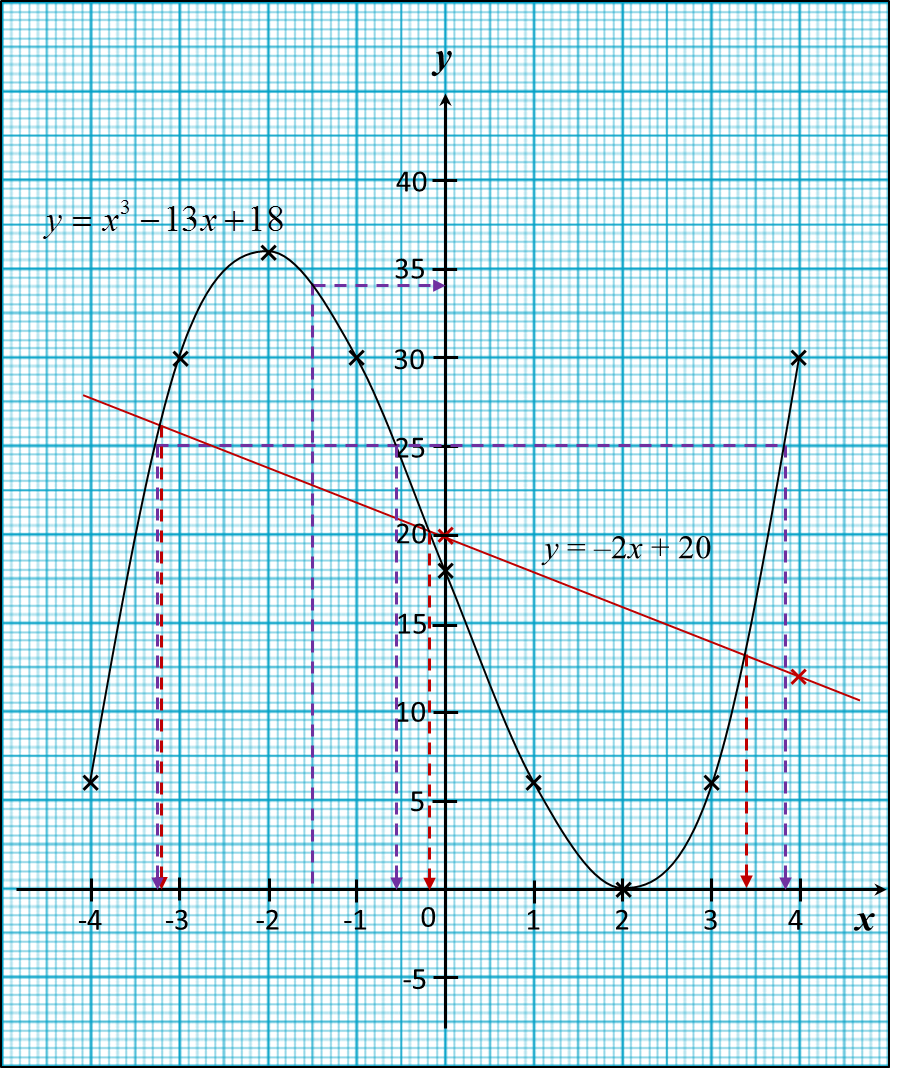# 2.5 Graph of Functions (II), SPM Paper 2 (Long Questions)

Question 9:
(a) Complete the table in the answer space for the equation y = 8 – 3x – 2x2 by writing down the values of y when x = –4 and x = 2.

(b) For this part of the question, use graph paper. You may use a flexible curve rule.
By using a scale of 2cm to 1 unit on the x-axis and 2cm to 5 units on the y-axis, draw the graph of y = 5 – 8x – 2x2 for –5 ≤ x ≤ 3 and –27 ≤ y ≤ 9.

(c) From your graph, find
(i) the value of y when x = –2.5,
(ii) the positive value of x when y = 16.

(d) Draw a suitable straight line on your graph to find the values of x which satisfy the equation 8 – 3x – 2x2 = 0 for –5 ≤ x ≤ 3 and –27 ≤ y ≤ 9.

 x –5 –4 –3.5 –2 –1 0 1 2 3 y –27 r –6 6 9 8 3 s –19
Calculate the value of r and s.

Solution:
(a)
y = 8 – 3x – 2x2
when x = –4,
r = 8 – 3(–4) – 2 (–4)2
= 8 + 12 – 32 = –12

when x = 2,
s = 8 – 3(2) – 2(2)2
= 8 – 6 – 8 = –6

(b)(c)
(i) From the graph, when x = 2.5, y = 2.5
(ii) From the graph, when y = 16, positive value of x = 2.8

(d)
y = 8 – 3x – 2x2 —– (1)
0 = 5 – 8x – 2x2  —– (2)
(1) – (2) : y = 3 + 5xy = 5x +3
The suitable straight line is y = 5x +3.

Determine the x-coordinates of the two points of intersection of the curve y = 8 – 3x – 2x2 and the straight line y = 5x +3.

 x –5 0 y = 5x + 3 22 3
From the graph, x = –4.5, 0.55.

Question 10:
(a) Complete the table in the answer space for the equation y = x3 – 13x + 18 by writing down the values of y when x = –2 and x = 3.

(b) For this part of the question, use graph paper. You may use a flexible curve rule.
By using a scale of 2cm to 1 unit on the x-axis and 2cm to 5 units on the y-axis, draw the graph of y = x3 – 13x + 18 for –4 ≤ x ≤ 4 and 0 ≤ y ≤ 40.

(c) From your graph, find
(i) the value of y when x = –1.5,
(ii) the value of x when y = 25.

(d) Draw a suitable straight line on your graph to find the values of x which satisfy the equation x3 – 11x – 2 = 0 for –4 ≤ x ≤ 4 and 0 ≤ y ≤ 40.Solution:
(a)
y = x3 – 13x + 18

when x = –2,
y = (–2)3 – 13(–2) + 18
= –8 + 26 + 18
= 36

when x = 3,
y = (3)3 – 13(3) + 18
= 27 – 39 + 18
= 6

(b)(c)
(i) From the graph, when x = –1.5, y = 34.
(ii) From the graph, when y = 25, x = –3.25, –0.55 and 3.85.

(d)
y = x3 – 13x + 18 —– (1)
0 = x3 – 11x – 2 —– (2)
(1) – (2) : y = –2x + 20

The suitable straight line is y = –2x + 20.
Determine the x-coordinates of the two points of intersection of the curve y = x3 – 13x + 18 and the straight line y = –2x + 20.From the graph, x = –3.2, –0.2 and 3.4.